Mathematical Symbols

(redirected from Table of mathematical symbols)
Also found in: Dictionary, Thesaurus, Wikipedia.

Mathematical Symbols

conventional designations used to represent mathematical concepts, propositions, and calculations. For example, √2 (the square root of 2) and 3 > 2 (3 is greater than 2).

The development of mathematical notation was closely connected with the overall development of the concepts and methods of mathematics. The first mathematical symbols, whose appearance apparently preceded writing, were those used to represent numbers. The most ancient systems of numeration—the Babylonian and Egyptian—appeared as early as 3500 B.C.

The first mathematical symbols for arbitrary quantities appeared much later (beginning in the fifth and fourth centuries B.C.) in Greece. Quantities (areas, volumes, angles) were rep-resented in the form of segments, and the products of two arbitrary like quantities were given in the form of a rectangle constructed on appropriate segments. In Euclid’s Elements (third century B.C.) quantities are designated by two letters—the initial and final letters of the corresponding segment—and occasionally by one letter. In Archimedes’ work (third century B.C.) the latter method became conventional. Such designation contained the possibilities for the development of literal calculation. However, literal calculation was not created in the mathematics of the classical period.

The rudiments of literal notation and calculation arose in the late Hellenistic era as a result of the liberation of algebra from geometric form. Diophantus (probably in the third century A.D.) wrote the unknown (x) and its power in the following symbols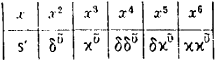υ̇—from the Greek term δúναμις (dynamis, “power”), which designated the square of an unknown quantity, and k̇υ, from the Greek kύβoς (kýbos, “cube”)]. Diophantus wrote the coefficients to the right of the unknown or its powers; for example, 3x5 was represented as δkῡγ̄(where γ̄ = 3). To indicate addition Diophantus put the terms alongside each other, and for subtraction he used the special symbol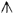. Diophantus indicated equality with the letter ι (from the Greek ïσoς [isos, “equal”]). For example, Diophantus would have written the equation

(x3 + 8x) – (5x2 + 1) = x

as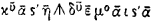(where ᾱ = 1, η̄ = 8, ε̄ = 5, and μ0ᾱ denotes that the unit ᾱ does not have a factor in the form of a power of the unknown).

Several centuries later the Hindus introduced various mathematical symbols for several unknowns (abbreviations of the names of colors, which designated unknowns), the square, the square root, and the subtrahend. Thus, the equation

3x2 + 10x – 8 = x2 + 1

would have had the following form in the notation of Brahmagupta (seventh century A.D.):

ya va 3 ya 10 ru 8

ya va 1 ya 0 ru 1

(ya [from yavat or tavat], unknown; va [from varga], a square; ru [fromrupa, a rupee coin], a free term; the dot over the number indicates the subtrahend).

The origin of modern algebraic notation dates from the 14th-17th centuries A.D.; it was brought about by advances in practical arithmetic and the study of equations. Mathematical symbols for some operations and for the powers of an unknown quantity appeared spontaneously in various countries. Many decades and even centuries passed before convenient notation was worked out. Thus, at the end of the 15th century N. Chuquet and L. Pacioli used the addition and subtraction symbols p and rh (from the Latin plus and minus), and German mathematicians introduced the modern symbol + (probably a contraction of the Latins) and –. As early as the 17th century about ten mathematical symbols for the multiplication operation could be found.

The mathematical symbols for an unknown quantity and its powers were also different. In the 16th century and at the beginning of the 17th more than ten symbols were in competition for the square of an unknown quantity alone, for example, ce (from census, a Latin term that served as a translation of the Greek dynamos), Q (from quadratum),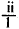A(2), 1

Ajj, aa, and a2. Thus, the Italian mathematician G. Cardano (J. Cardan; 1545) would have written the equation

x3 + 5x = 12

as

I. cubis p̄. 5. positionibus aequantur 12

the German mathematician M. Stifel (1544) as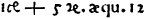the Italian mathematician R. Bombelli (1572) as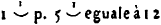the French mathematician F. Vieta (1591) as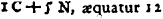and the English mathematician T. Harriot (1631) as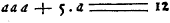The equals sign and brackets (R. Bombelli, 1550), parentheses (N. Tartaglia, 1556), and braces (F. Vieta, 1593) came into use in the 16th century and early 17th. In the 16th century the notation of fractions assumed its modern form.

Vieta’s introduction (in 1591) of mathematical notation for arbitrary constants in the form of the capitalized consonants of the Latin alphabet B and D was a significant step forward in the development of mathematical notation. This enabled him for the first time to write algebraic equations with arbitrary coefficients and to manipulate them. Vieta represented unknowns with capitalized vowels such as A and E. For example, in our symbols Vieta’s notation

A cubus + B plano in A3, æquatur Dsolido

(cubus, “cube”; planus, “flat,” that is, B is a two-dimensional quantity; and solidus, “corporeal” [three-dimensional]; the dimensionality was marked so that all terms were similar) would appear as

x3 + 3bx = d

Vieta was the originator of algebraic formulas. R. Descartes (1637) gave algebraic symbols their modern form, designating unknowns with the last letters of the Latin alphabet x, y, z and arbitrary given quantities with the initial letters a, b, c. He was also responsible for the current notation of powers. Descartes’s symbols had a great advantage over all previous symbols and soon gained universal recognition.

The further development of mathematical symbols was closely connected with the birth of the analysis of infinitesimals, for which the foundation for the development of a notation had already been largely prepared in algebra.

In his method of fluxions and fluents (1666 and subsequent years), I. Newton introduced symbols for the successive fluxions (derivatives) of a quantity x in the form ẋ ẍ ẋ̈ and for the infinitely small increment, o. Somewhat earlier J. Wallis (1655) proposed the infinity sign ∞.

G. von Leibniz was the originator of the modern notation for differential and integral calculus. In particular, he was responsible for the currently used mathematical symbols for differentials

dx, d2x, d3x

and the integral

ydx

L. Euler deserves great credit for the development of the notation of modern mathematics. He introduced (1734) the first symbol for a variable operation into general use, namely the function symbol f(x) (from the Latin functio). After Euler’s work the notation for many individual functions, such as trigonometric functions, assumed a standard form. Euler was also responsible for the designations for the constants e (the base of natural logarithms, 1736) and π (probably from the Greek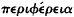[periphéreia, “perimeter,” “periphery,” 1736]) and for the imaginary unit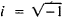(from the Frenchimaginaire, 1777, published in 1794).

The role of mathematical notation increased in the 19th century. At this time the symbols for an absolute value ǀxǀ (K. Weierstrass, 1841), the vector r̄ (A. Cauchy, 1853), and the determinant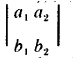(A. Cayley, 1841), among others, appeared. Many theories that arose in the 19th century, such as tensor calculus, could not be developed without appropriate notations.

In addition to this process of the standardization of mathematical notation, mathematical symbols used by some authors only within a particular investigation can be found with extreme frequency in the modern literature.

From the standpoint of mathematical logic, the following main groups of mathematical symbols can be noted: (A) symbols designating objects, (B) symbols designating operations, and (C) symbols designating relations. For example, the symbols 1, 2, 3, 4 represent numbers, that is, the objects studied by arithmetic. The symbol for the operation of addition + does not in itself represent any object. The plus sign gains subjective content only when it is indicated which numbers are being added: the notation 1 -j- 3 represents the number 4. The symbol > (greater than) indicates a relation between numbers. The relation symbol gains quite definite meaning only when it is indicated between the objects whose relation is considered. A fourth group borders on these three main groups of mathematical symbols: (D) auxiliary symbols that establish the sequence in which primary symbols are combined. Parentheses, which indicate the sequence in which operations are to be performed, provide an adequate idea of such symbols.

The symbols of each of the three groups A, B, and C are of two types: (1) individual symbols for fully defined objects, operations, and relations and (2) general symbols for “variable,” or “unknown,” objects, operations, and relations.

The following may serve as examples of symbols of the first type (see also Table 1):

(A1) The designations for the natural numbers 1, 2, 3, 4, 5, 6, 7, 8, 9; the transcendental numbers e and π and the imaginary number i.

(B1) The symbols for arithmetic operations +, −, ·, ×, ÷; root extraction 11√; differentiation d/dx, and the sum (union υ and the product (intersection) ∩ of sets. The symbols for the individual functions, such as sin, tan, and log, also fall into this category.

(C1) The symbols for equality and inequality =, >, <, ≠; parallelism ∥; perpendicularity ⊥; and membership in some set ∈ and inclusion of one set in another ⊂.

Symbols of the second type represent arbitrary objects, operations, and relations of a specific class or objects, operations, and relations that conform to some previously stipulated conditions. For example, in the notation for the identity (a + b) (a - b) = a2 - b2 the letters a and b designate arbitrary numbers. In studying the functional relation y = x2, the letters x and y are arbitrary numbers connected by an assigned relation. In the solution of the equation

x2 − 1 = 0

x denotes any number that satisfies this equation (as a result of solving this equation we learn that only two possible values, +1 and −1, conform to this condition).

From the logical point of view, it is legitimate to call general symbols of this type variable symbols, as is common in mathematical logic, without fearing that the “range” of a variable may prove to consist of a single entity or even to be “empty” (for example, in the case of equations that have no solutions). The following may serve as further examples of this type of symbol:

(A2) The designation of points, lines, planes, and more complex geometric figures with letters in geometry.

(B2) The symbols f, F, ϕ for functions and the symbols of operational calculus, where the one letter L is used to represent, for example, an arbitrary operator of the type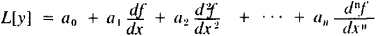The symbols for “variable relations” are less widely used and find application only in mathematical logic and in comparatively abstract, primarily axiomatic, mathematical investigations.

REFERENCE

Cajori, F. A History of Mathematical Notations, vols. 1–2. Chicago, 1928–29.
Site: Follow: Share:
Open / Close